# Computer Oragnization Flipflops

Jul. 5, 2013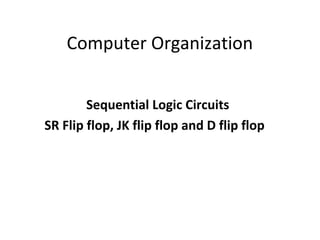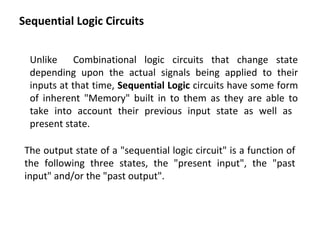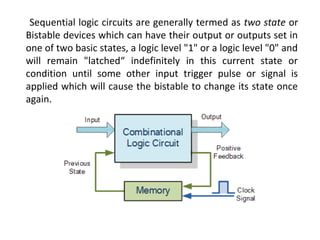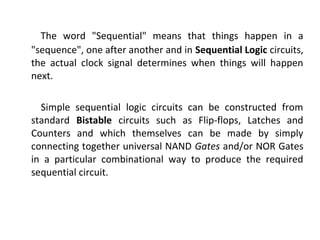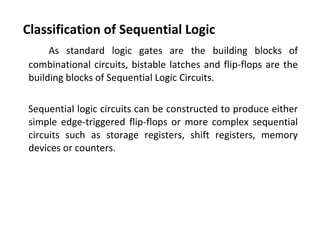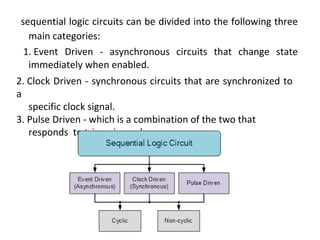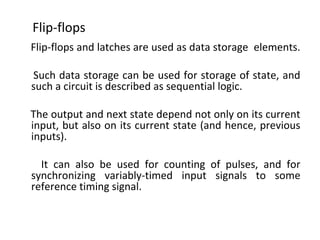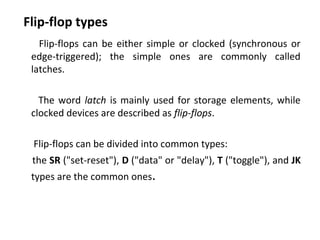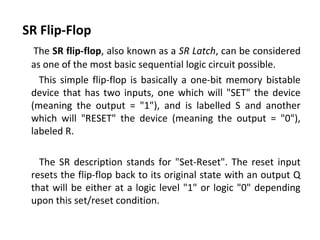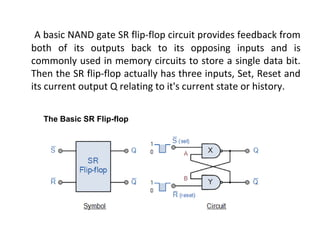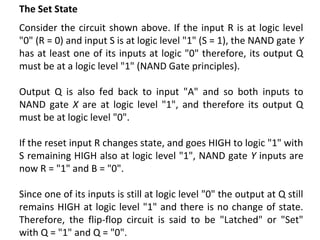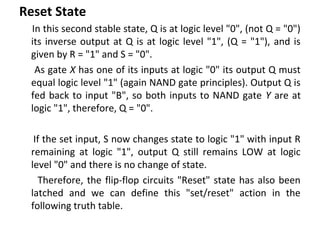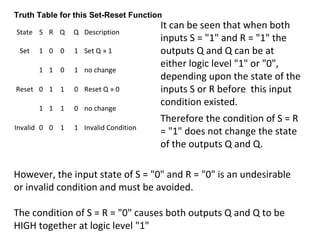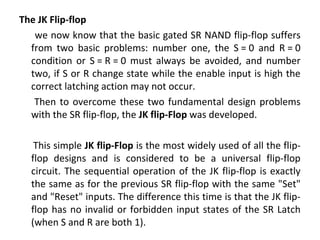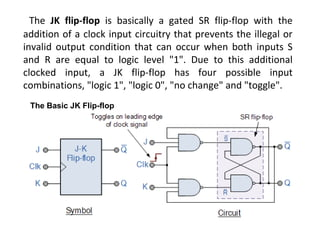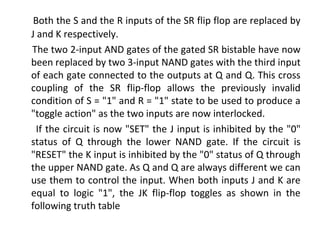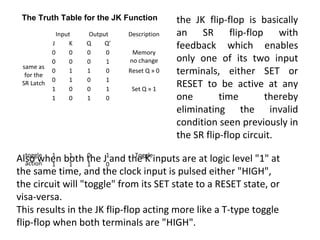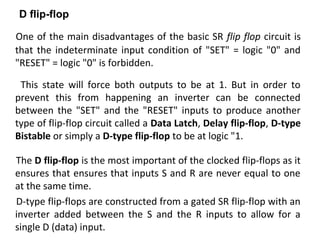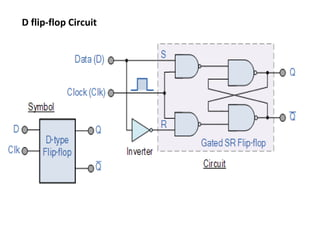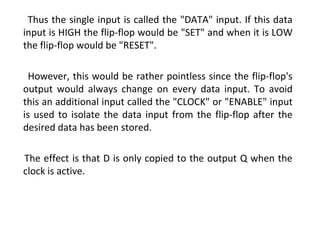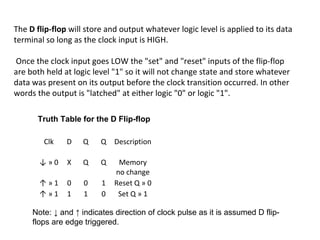1 of 22

### Computer Oragnization Flipflops

• 1. Computer Organization Sequential Logic Circuits SR Flip flop, JK flip flop and D flip flop
• 2. Sequential Logic Circuits Unlike Combinational logic circuits that change state depending upon the actual signals being applied to their inputs at that time, Sequential Logic circuits have some form of inherent "Memory" built in to them as they are able to take into account their previous input state as well as present state. The output state of a "sequential logic circuit" is a function of the following three states, the "present input", the "past input" and/or the "past output".
• 3. Sequential logic circuits are generally termed as two state or Bistable devices which can have their output or outputs set in one of two basic states, a logic level "1" or a logic level "0" and will remain "latched“ indefinitely in this current state or condition until some other input trigger pulse or signal is applied which will cause the bistable to change its state once again.
• 4. The word "Sequential" means that things happen in a "sequence", one after another and in Sequential Logic circuits, the actual clock signal determines when things will happen next. Simple sequential logic circuits can be constructed from standard Bistable circuits such as Flip-flops, Latches and Counters and which themselves can be made by simply connecting together universal NAND Gates and/or NOR Gates in a particular combinational way to produce the required sequential circuit.
• 5. Classification of Sequential Logic As standard logic gates are the building blocks of combinational circuits, bistable latches and flip-flops are the building blocks of Sequential Logic Circuits. Sequential logic circuits can be constructed to produce either simple edge-triggered flip-flops or more complex sequential circuits such as storage registers, shift registers, memory devices or counters.
• 6. sequential logic circuits can be divided into the following three main categories: 1. Event Driven - asynchronous circuits that change state immediately when enabled. 2. Clock Driven - synchronous circuits that are synchronized to a specific clock signal. 3. Pulse Driven - which is a combination of the two that responds to triggering pulses.
• 7. Flip-flops and latches are used as data storage elements. Such data storage can be used for storage of state, and such a circuit is described as sequential logic. The output and next state depend not only on its current input, but also on its current state (and hence, previous inputs). It can also be used for counting of pulses, and for synchronizing variably-timed input signals to some reference timing signal. Flip-flops
• 8. Flip-flop types Flip-flops can be either simple or clocked (synchronous or edge-triggered); the simple ones are commonly called latches. The word latch is mainly used for storage elements, while clocked devices are described as flip-flops. Flip-flops can be divided into common types: the SR ("set-reset"), D ("data" or "delay"), T ("toggle"), and JK types are the common ones.
• 9. SR Flip-Flop The SR flip-flop, also known as a SR Latch, can be considered as one of the most basic sequential logic circuit possible. This simple flip-flop is basically a one-bit memory bistable device that has two inputs, one which will "SET" the device (meaning the output = "1"), and is labelled S and another which will "RESET" the device (meaning the output = "0"), labeled R. The SR description stands for "Set-Reset". The reset input resets the flip-flop back to its original state with an output Q that will be either at a logic level "1" or logic "0" depending upon this set/reset condition.
• 10. A basic NAND gate SR flip-flop circuit provides feedback from both of its outputs back to its opposing inputs and is commonly used in memory circuits to store a single data bit. Then the SR flip-flop actually has three inputs, Set, Reset and its current output Q relating to it's current state or history. The Basic SR Flip-flop
• 11. The Set State Consider the circuit shown above. If the input R is at logic level "0" (R = 0) and input S is at logic level "1" (S = 1), the NAND gate Y has at least one of its inputs at logic "0" therefore, its output Q must be at a logic level "1" (NAND Gate principles). Output Q is also fed back to input "A" and so both inputs to NAND gate X are at logic level "1", and therefore its output Q must be at logic level "0". If the reset input R changes state, and goes HIGH to logic "1" with S remaining HIGH also at logic level "1", NAND gate Y inputs are now R = "1" and B = "0". Since one of its inputs is still at logic level "0" the output at Q still remains HIGH at logic level "1" and there is no change of state. Therefore, the flip-flop circuit is said to be "Latched" or "Set" with Q = "1" and Q = "0".
• 12. Reset State In this second stable state, Q is at logic level "0", (not Q = "0") its inverse output at Q is at logic level "1", (Q = "1"), and is given by R = "1" and S = "0". As gate X has one of its inputs at logic "0" its output Q must equal logic level "1" (again NAND gate principles). Output Q is fed back to input "B", so both inputs to NAND gate Y are at logic "1", therefore, Q = "0". If the set input, S now changes state to logic "1" with input R remaining at logic "1", output Q still remains LOW at logic level "0" and there is no change of state. Therefore, the flip-flop circuits "Reset" state has also been latched and we can define this "set/reset" action in the following truth table.
• 13. State S R Q Q Description Set 1 0 0 1 Set Q » 1 1 1 0 1 no change Reset 0 1 1 0 Reset Q » 0 1 1 1 0 no change Invalid 0 0 1 1 Invalid Condition Truth Table for this Set-Reset Function It can be seen that when both inputs S = "1" and R = "1" the outputs Q and Q can be at either logic level "1" or "0", depending upon the state of the inputs S or R before this input condition existed. Therefore the condition of S = R = "1" does not change the state of the outputs Q and Q. However, the input state of S = "0" and R = "0" is an undesirable or invalid condition and must be avoided. The condition of S = R = "0" causes both outputs Q and Q to be HIGH together at logic level "1"
• 14. The JK Flip-flop we now know that the basic gated SR NAND flip-flop suffers from two basic problems: number one, the S = 0 and R = 0 condition or S = R = 0 must always be avoided, and number two, if S or R change state while the enable input is high the correct latching action may not occur. Then to overcome these two fundamental design problems with the SR flip-flop, the JK flip-Flop was developed. This simple JK flip-Flop is the most widely used of all the flip- flop designs and is considered to be a universal flip-flop circuit. The sequential operation of the JK flip-flop is exactly the same as for the previous SR flip-flop with the same "Set" and "Reset" inputs. The difference this time is that the JK flip- flop has no invalid or forbidden input states of the SR Latch (when S and R are both 1).
• 15. The JK flip-flop is basically a gated SR flip-flop with the addition of a clock input circuitry that prevents the illegal or invalid output condition that can occur when both inputs S and R are equal to logic level "1". Due to this additional clocked input, a JK flip-flop has four possible input combinations, "logic 1", "logic 0", "no change" and "toggle". The Basic JK Flip-flop
• 16. Both the S and the R inputs of the SR flip flop are replaced by J and K respectively. The two 2-input AND gates of the gated SR bistable have now been replaced by two 3-input NAND gates with the third input of each gate connected to the outputs at Q and Q. This cross coupling of the SR flip-flop allows the previously invalid condition of S = "1" and R = "1" state to be used to produce a "toggle action" as the two inputs are now interlocked. If the circuit is now "SET" the J input is inhibited by the "0" status of Q through the lower NAND gate. If the circuit is "RESET" the K input is inhibited by the "0" status of Q through the upper NAND gate. As Q and Q are always different we can use them to control the input. When both inputs J and K are equal to logic "1", the JK flip-flop toggles as shown in the following truth table
• 17. same as for the SR Latch Input Output Description J K Q Q’ 0 0 0 0 Memory no change0 0 0 1 0 1 1 0 Reset Q » 0 0 1 0 1 1 0 0 1 Set Q » 1 1 0 1 0 toggle action 1 1 0 1 Toggle 1 1 1 0 The Truth Table for the JK Function the JK flip-flop is basically an SR flip-flop with feedback which enables only one of its two input terminals, either SET or RESET to be active at any one time thereby eliminating the invalid condition seen previously in the SR flip-flop circuit. Also when both the J and the K inputs are at logic level "1" at the same time, and the clock input is pulsed either "HIGH", the circuit will "toggle" from its SET state to a RESET state, or visa-versa. This results in the JK flip-flop acting more like a T-type toggle flip-flop when both terminals are "HIGH".
• 18. One of the main disadvantages of the basic SR flip flop circuit is that the indeterminate input condition of "SET" = logic "0" and "RESET" = logic "0" is forbidden. This state will force both outputs to be at 1. But in order to prevent this from happening an inverter can be connected between the "SET" and the "RESET" inputs to produce another type of flip-flop circuit called a Data Latch, Delay flip-flop, D-type Bistable or simply a D-type flip-flop to be at logic "1. The D flip-flop is the most important of the clocked flip-flops as it ensures that ensures that inputs S and R are never equal to one at the same time. D-type flip-flops are constructed from a gated SR flip-flop with an inverter added between the S and the R inputs to allow for a single D (data) input. D flip-flop
• 20. Thus the single input is called the "DATA" input. If this data input is HIGH the flip-flop would be "SET" and when it is LOW the flip-flop would be "RESET". However, this would be rather pointless since the flip-flop's output would always change on every data input. To avoid this an additional input called the "CLOCK" or "ENABLE" input is used to isolate the data input from the flip-flop after the desired data has been stored. The effect is that D is only copied to the output Q when the clock is active.
• 21. Clk D Q Q Description ↓ » 0 X Q Q Memory no change ↑ » 1 0 0 1 Reset Q » 0 ↑ » 1 1 1 0 Set Q » 1 Truth Table for the D Flip-flop Note: ↓ and ↑ indicates direction of clock pulse as it is assumed D flip- flops are edge triggered. The D flip-flop will store and output whatever logic level is applied to its data terminal so long as the clock input is HIGH. Once the clock input goes LOW the "set" and "reset" inputs of the flip-flop are both held at logic level "1" so it will not change state and store whatever data was present on its output before the clock transition occurred. In other words the output is "latched" at either logic "0" or logic "1".